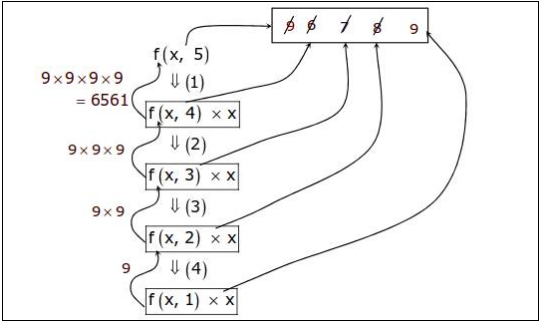##### What is the return value of f(p, p) if the value of p is initialized to 5 before the call? Note that the first parameter is passed by reference, whereas the second parameter is passed by value?

1) What is the return value of f(p, p) if the value of p is initialized to 5 before the call? Note that the first parameter is passed by reference, whereas the second parameter is passed by value.

int f(int &x, int c) {
c  = c - 1;
if (c == 0) return 1;
x = x + 1;
return f(x, c) * x;
} 

(A) 3024
(B) 6561
(C) 55440
(D) 161051

Sumit Verma
21 Jun 2017 01:50 pm

(B) 6561

it can be simply seen by below figure :Also ,  the catch here is , x is passed by reference , so each loop will change the value of x.

nandini
21 Jun 2017 12:59 pm

Sir, from where this 9 came ?

Sumit Verma
21 Jun 2017 01:50 pm

@nandini1508 In this code, there will be 4 recursive calls with parameters (6,4), (7,3), (8,2) and (9,1). The last call returns 1. But due to pass by reference, x in all the previous functions is now 9. Hence, the value returned by f(p,p) will be 9 * 9 * 9 * 9 * 1 = 6561.